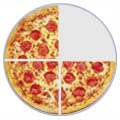# Adding and Subtracting Mixed FractionsQuick Definition: A Mixed Fraction is a whole number and a fraction combined, such as  1 3 4 1 3/4 (one and three-quarters)

To make it easy to add and subtract them, just convert to Improper Fractions first:Quick Definition: An Improper fraction has a top number larger than or equal to the bottom number, such as  7 4  or  4 3 (It is "top-heavy") 7/4 (seven-fourths or seven-quarters)

Can you see that 134 is the same as 74 ?

I find this is the best way to add mixed fractions:

(You may like to read how to Convert from or to Mixed Fractions)

### Example: What is  2 3 4  +  3 1 2   ?

Convert to Improper Fractions:

2 3 4  =  11 4

3 1 2  =  7 2

Common denominator of 4:

11 4  stays as  11 4

7 2  becomes  14 4
(by multiplying top and bottom by 2)

11 4  +  14 4  =  25 4

Convert back to Mixed Fractions:

25 4  =  6 1 4

When you get more experience you can do it faster like this example:

### Example: What is  3 5 8  +  1 3 4

Convert them to improper fractions:

3 5 8  =  29 8
1 3 4  =  7 4

Make same denominator:  7 4  becomes  14 8  (by multiplying top and bottom by 2)

29 8  +  14 8  =  43 8  =  5 3 8

## Subtracting Mixed Fractions

### Example: What is  15 3 4  −  8 5 6  ?

Convert to Improper Fractions:

15 3 4  =  63 4

8 5 6  =  53 6

Common denominator of 12:

63 4  becomes  189 12

53 6  becomes  106 12

Now Subtract:

189 12  −  106 12  =  83 12

Convert back to Mixed Fractions:

83 12  =  6 11 12

Hard Question